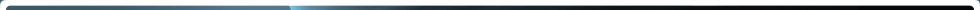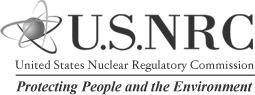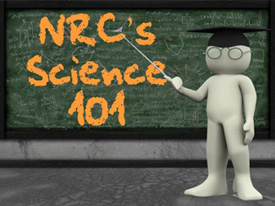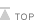Home > NRC Library > Basic References > The Student Corner > Science 101 > What are Quantities and Measurement?

# What are Quantities and Measurement?

Printable Version

## The Nuclear Regulatory Commission's Science 101: What are Quantities and Measurement?Scientists use measurements made in various units of measure, particularly meters, grams and liters. Where did these units of measure come from and how do they relate to one another?

Quantities are characteristics or properties we are trying to measure, such as the length of an object. Units of measure are how we express measurements of quantities. For length, the unit we would use in science is meters. A unit is really only a particular amount of some quantity used as a reference point for measurements of that quantity. Put differently, units of measure are chosen and accepted by the people who use them.

Often, units of measure were agreed upon many years ago. One meter is as long as it is because that's what scientists agreed to use as a base unit for length. A meter could have been some other length. For the sake of establishing well-defined and easily-accessible units of measure, the General Conference of Weights and Measures (a collection of scientists from multiple countries) created in 1960 the Sytème International d'Unités. This system relies upon the base units of measure listed in the following table.

Quantity Unit Abbreviation
Length Meter m
Mass Kilogram kg
Time Second s
Thermodynamic Temperature Kelvin K
Amount of Substance Mole mol
Electric Current Ampere AOften times, however, the base unit of measure can be either too large or too small to be useful in describing a particular measurement. For example, while we could talk about the distances between cities in meters, we would be using very large (and, as such, cumbersome) numbers. For example, from Portland, Maine, to St. Louis, Missouri, is 2,060,000 m or 2,060 km. Similarly, if we are talking about the size of atoms, the basic building blocks of matter, speaking in terms of meters would be difficult as the diameter of a hydrogen atom is only 0.000000000120m. So, instead, for the sake of convenience, we often use prefixes to modify the size of the base unit.

This table lists a number of such prefixes using meter for the base. It also lays out conversion factors, ratios that can be used to convert one unit to another.

Prefix Abbreviation Exponential
Multiplier
Meaning Example using Length
giga G 109 1000000000 1 gigameter (Gm) = 1000000000m
mega M 106 1000000 1 megameter (Mm) = 1000000m
kilo k 103 1000 1 kilometer (km) = 1000m
hecto h 102 100 1 hectometer (hm) = 100m
deka da 101 10 1 dekameter (dam) = 10m
1 1 meter (m)
deci d 10-1 1/10 1 decimeter (dm) = -0.1m
centi c 10-2 1/100 1 centimeter (cm) = 0.01m
milli m 10-3 1/1000 1 millimeter (mm) = 0.001m
micro µ 10-6 1/1000000 1 micrometer (µm) = 0.000001m
nano n 10-9 1/1000000000 1 nanometer (nm) = 0.000000001m
pico p 10-12 1/1000000000000 1 picometer (pm) = 0.000000000001m

The U.S. Nuclear Regulatory Commission is an independent federal government agency responsible for regulating the commercial use of nuclear materials. This document is free of copyright and can be reproduced for educational purposes.Page Last Reviewed/Updated Friday, October 19, 2018

#### Home